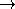## CGAL::min_element_if

### Definition

The function min_element_if computes the minimum among the elements of a range which satisfy a certain predicate. It is modeled after the STL function min_element.

#include <CGAL/algorithm.h>

template < class ForwardIterator, class Predicate >
ForwardIterator
 min_element_if ( ForwardIterator first, ForwardIterator last, Predicate pred)
returns an iterator referring to the minimal element among those satifying the predicate pred in the range [first, last). The ordering is defined by the operator< on VT where VT is the value type of ForwardIterator.
Requirement: pred is an unary function object: VTbool.

template < class ForwardIterator, class Compare, class Predicate >
ForwardIterator
 min_element_if ( ForwardIterator first, ForwardIterator last, Compare comp, Predicate pred)
return an iterator referring to the minimal element among those satifying the predicate pred in the range [first, last). The ordering is defined by comp.
Requirement: comp is a binary function object: VT  ×  VTbool where VT is the value type of ForwardIterator. pred is an unary function object: VTbool.

CGAL::max_element_if
CGAL::min_max_element

### Example

The following example program computes the minimal odd element of the sequence (3,5,2). Hence the output is min_odd = 3.

```#include <CGAL/algorithm.h>
#include <CGAL/function_objects.h>
#include <vector>
#include <iostream>
#include <functional>

using std::vector;
using std::cout;
using std::endl;
using std::modulus;
using std::greater;
using std::bind2nd;
using CGAL::compose1_1;
using CGAL::min_element_if;

int main()
{
vector< int > v;
v.push_back(3);
v.push_back(5);
v.push_back(2);
cout << "min_odd = "
<< *min_element_if(v.begin(),
v.end(),
compose1_1(bind2nd(greater< int >(), 0),
bind2nd(modulus< int >(), 2)))
<< endl;
return 0;
}
```